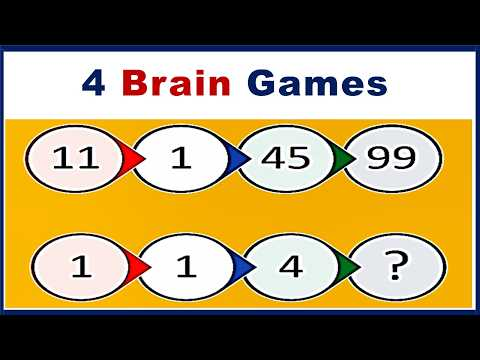# Math Puzzles with Answers | Number Pattern Logictricky maths puzzles with answers, picture maths puzzles with answers, maths puzzles with solutions, math brain teasers with answers, challenging math riddles with answers, math puzzles for adults

4 Math puzzles with answers are based on number pattern logic in this video. Find the logic in given numbers to get the missing number. Are you genius, then solve hard and difficult 4 math new puzzles challenge using your intelligence. These are tricky questions and Brainteaser for mental exercise. Educational video tutorial by G K Agrawal 239. The logic of the pattern of the numbers is given below.

Quiz 1– Multiply number with 1 smaller number and then add 1. For example, Number 9 will be multiplied by 8 and then add 1, you get 73

Quiz 2– Take the square of the 1st number, then subtract the second number, then add 3.

Quiz 3– Add double of the 2nd number in the 1st number, then subtract 3rd number, then add 1.

Quiz 4– Add 1st & 2nd number, then take the square of it, then subtract 3rd number,

Math Quiz for genius, 4 new puzzles with the answer in video Number pattern logic puzzles. Riddles, Math Puzzle. 4 math puzzles with answers. Difficult math puzzles. Hard math puzzles. Trick question. IQ test. Riddles with answers. पहेलियां

If you liked this video, please go to Youtube and subscribe to this channel.

Official Website

Share